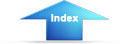Custom SearchIntegrated Publishing's Mathematics Sub-CategoryPre-Calculus and Intro to ProbabilityChapter 1 - Numbers and Sets

Definitions and symbols

Chapter 2 - Positive Integers

Chapter 3 - Signed Numbers

Chapter 4 - Common Fractions

Chapter 5 - Decimals

Chapter 6 - Percentage and Measurement

Chapter 7 - Exponents and Radicals

Chapter 8 - Logarithms and the slide rule

Chapter 9 - Fundamentals of Algebra

Chapter 10 - Factoring Polynomials

Chapter 11 - Linear Equations in one variable

Chapter 12 - Linear equations in two variables

Chapter 13 - Ratio, Proportion, and Variation

Chapter 14 - Dependence, Functions, and Formulas

Chapter 15 - Complex Numbers

Chapter 16 - Quadratic equations in one variable

Chapter 17 - Plane Figures

Chapter 18 - Geometric constructions and solid figures

Chapter 19 - Numerical TrigonometryIntegrated Publishing, Inc. - A (SDVOSB) Service Disabled Veteran Owned Small Business